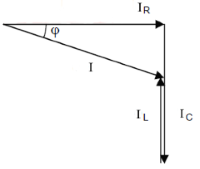# RLC parallel circuit calculation

Calculator and formulas for calculating the voltage and power of an RLC parallel circuit

## Calculate RLC parallel circuit

The calculator calculates the voltages, powers, currents, impedance and reactance in the parallel circuit of a resistor of a inductor and a capacitor.

Calculate RLC parallel circuit

 Input Inductor L H mH µH nH Capacitor C F mF µF nF pF Frequency f Hz kHz MHz GHz Resistor R mΩ Ω kΩ MΩ Voltage U mV V kV Decimal places 0 1 2 3 4 6 8 10 Result Reactance XL Reactance XC Impedance Z Inductor current IL Capacitor curent IC Resistor current UR Current I Active power P Reactive power QL Reactive power QC Apparent power S Phase angle φ

## Formulas for RLC parallel circuit

The total resistance of the RLC series circuit in an AC circuit is as Impedance Z denotes. Ohm's law applies to the entire circuit.

• Current and voltage are in phase at the ohmic resistance.

• At the inductive reactance of the coil, the voltage leads the current by + 90 °.

• At the capacitive reactance of the capacitor, the voltage lags the current by -90 °.

• Therefore UL and UC are out of phase by 180 °.

The total current I is the sum of the geometrically added partial currents.

For this purpose, the current at the resistor forms the leg of a right triangle. The other cathede is the difference between the currents IL and IC , since these are out of phase. The hypotenuse corresponds to the total current I.

The resulting triangle is called the current triangle or vector diagram of the currents.

### Current triangle

 $$\displaystyle I=\sqrt{ {I_R}^2 + (I_C-I_L)^2}$$

 $$\displaystyle I_L$$ Current through the inductor $$\displaystyle I_C$$ Current through the capacitor $$\displaystyle I_R$$ Current through the resistor $$\displaystyle I$$ Total current### Conductance triangle

 $$\displaystyle Y=\sqrt{G^2 + (B_L-B_C)^2}$$

 $$\displaystyle Y$$ Admittance $$\displaystyle G$$ Real conductance $$\displaystyle B_L$$ Inductive susceptance $$\displaystyle B_C$$ Capacitive susceptance

### Power triangle

 $$\displaystyle S=\sqrt{P^2 + (Q_L-Q_C)^2}$$

 $$\displaystyle P$$ Real power $$\displaystyle S$$ Apparent power $$\displaystyle Q_L$$ Inductive reactive power $$\displaystyle Q_C$$ Capacitive reactive power

### More formulas

#### Current

$$\displaystyle I=\frac{U}{Z}$$ $$\displaystyle I_R=\frac{U}{R}$$ $$\displaystyle I_L=\frac{U}{X_L}$$ $$\displaystyle I_C=\frac{U}{X_C}$$

#### Resistor

$$\displaystyle X_L=2π · f · L$$ $$\displaystyle X_C=\frac{1}{2π · f · C}$$

#### Power

$$\displaystyle P=I·U_R$$ $$\displaystyle Q_L=I·U_L$$ $$\displaystyle Q_C=I·U_C$$

#### Phase

$$\displaystyle φ = acos\left(\frac{Z}{R}\right)$$

 Is this page helpful?             Thank you for your feedback! Sorry about that How can we improve it?